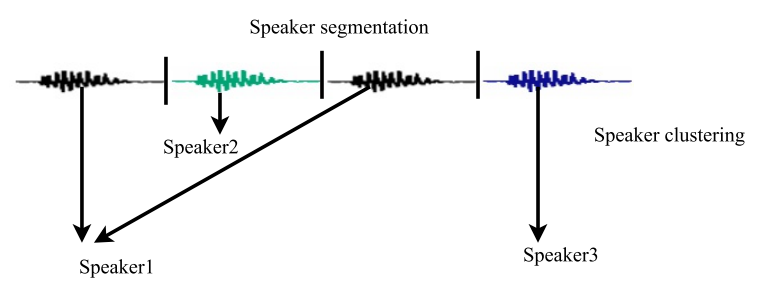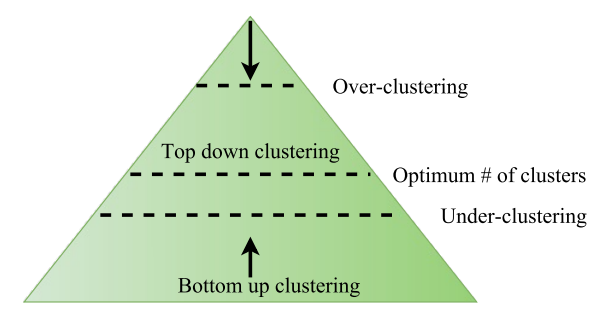# Speaker Diarization

### 1. Introduction to Speaker Diarization

Speaker diarization is the process of segmenting and clustering a speech recording into homogeneous regions and answers the question “who spoke when” without any prior knowledge about the speakers. A typical diarization system performs three basic tasks. Firstly, it discriminates speech segments from the non-speech ones. Secondly, it detects speaker change points to segment the audio data. Finally, it groups these segmented regions into speaker homogeneous clusters.An overview of a speaker diarization system.

Although there are many different approaches to perform speaker diarization, most of them follow the following scheme:

Feature extraction: It extracts specific information from the audio signal and allows subsequent speaker modeling and classification. The extracted features should ideally maximize inter-speaker variability and minimize intra-speaker variability, and represent the relevant information.

Speaker segmentation: It partitions the audio data into acoustically homogeneous segments according to speaker identities. It detects all boundary locations within each speech region that corresponds to speaker change points which are subsequently used for speaker clustering.

Speaker clustering: Speaker clustering groups speech segments that belong to a particular speaker. It has two major categories based on its processing requirements. Its two main categories are online and offline speaker clustering. In the former, speech segments are merged or split in consecutive iterations until the optimum number of speakers is acquired. Since the entire speech file is available before decision making in the later, it provides better results more than the online speaker clustering. The most widely used and popular technique for speaker clustering is Agglomerative Hierarchical Clustering (AHC). AHC builds a hierarchy of clusters, that shows the relations between speech segments, and merges speech segments based on similarity. AHC approaches can be classified into bottom-up and top-down clustering.

Two items need to be defined in both bottom-up and top-down clustering:

1. A distance between speech segments to determine acoustic similarity. The distance metric is used to decide whether or not two clusters must be merged (bottom-up clustering) or split (top-down clustering).

2. A stopping criterion to determine when the optimal number of clusters (speakers) is reached.

Bottom-up (Agglomerative): It starts from a large number of speech segments and merges the closest speech segments iteratively until a stopping criterion is met. This technique is the most widely used in speaker diarization since it is directly applied on the output of speech segments from speaker segmentation. A matrix of distances between every possible pair of clusters is computed and the pair with highest BIC value is merged. Then, the merged clusters are removed from the distance matrix. Finally, the distance matrix table is updated using the distances between the new merged cluster and all remaining clusters. This process is done iteratively until the stopping criterion is met or all pairs have a BIC value less than zero .

Top-down: Top-down Hierarchical Clustering methods start from a small number of clusters, usually a single cluster that contains several speech segments, and the initial clusters are split iteratively until a stopping criterion is met. It is not as widely used as the bottom-up clustering.Bottom-Up and Top-down approaches to clustering

### 2. Approaches to Speaker Diarization

This section describes some of the state-of-the-art speaker diarization systems.

HMM/GMM based speaker diarization system: Each speaker is represented by a state of an HMM and the state emission probabilities are modeled using GMMs. The initial clustering is performed initially by partitioning the audio signal equally which generates a set of segments { $$s_i$$ }. Let  $$c_i$$ represent  $$i^{th}$$ speaker cluster,  $$b_i$$ represent the emission probability of cluster  $$c_i$$ and  $$f_t$$ denote a given feature vector at time $$t$$ . Then, the log-likelihood  $$logb_i(s_t)$$ of the feature ft for cluster  $$c_i$$ is calculated as follows:

$logb_i(s_t)=log \sum_{(r)} {w}^r_i N (f_i,{\mu}^r_i,\sum_{(i)}^r)$

where  $$N()$$ is a Gaussian pdf and  $${w}^r_i, {\mu}^r_i,\sum_{(i)}^r)$$ are the weights, means and covariance matrices of the  $$r^{th}$$ Gaussian mixture component of cluster  $$c_i$$ , respectively.

The agglomerative hierarchical clustering starts by overestimating the number of clusters. At each iteration, the clusters that are most similar are merged based on the BIC distance. The distance measure is based on modified delta Bayesian information criterion [Ajmera and Wooters, 2003]. The modified BIC distance does not take into account the penalty term that corresponds to the number of free parameters of a multivariate Gaussian distribution and is expressed as:

$\Delta BIC (c_i,c_j)= log \sum_{f_t \in ( {ci \; \cup \; c_j})} log b_{ij}(f_t) - log \sum_{f_t \in ci} log b_{i}(f_t) - log \sum_{f_t \in cj} log b_{j}(f_t)$

where  $$b_{ij}$$ is the probability distribution of the combined clusters  $$c_i$$ and  $$c_j.$$

The clusters that produce the highest BIC score are merged at each iteration. A minimum duration of speech segments is normally constrained for each class to prevent decoding short segments. The number of clusters is reduced at each iteration. When the maximum  $$\Delta BIC$$

distance among these clusters is less than threshold value 0, the speaker diarization system stops and outputs the hypothesis.

Factor analysis techniques: Factor analysis techniques which are the state of the art in speaker recognition have recently been successfully used in speaker diarization. The speech clusters are first represented by i-vectors and the successive clustering stages are performed based on i-vector modeling. The use of factor analysis technique to model speech segments reduces the dimension of the feature vector by retaining most of the relevant information. Once the speech clusters are represented by i-vectors, cosine-distance and PLDA scoring techniques can be applied to decide if two clusters belong to the same or different speaker(s).

Deep learning approaches: Speaker diarization is crucial for many speech technologies in the presence of multiple speakers, but most of the current methods that employ i-vector clustering for short segments of speech are potentially too cumbersome and costly for the front-end role. Thus, it has been proposed by Daniel Povey an alternative approach for learning representations via deep neural networks to remove the i-vector extraction process from the pipeline entirely. The proposed architecture simultaneously learns a fixed-dimensional embedding for acoustic segments of variable length and a scoring function for measuring the likelihood that the segments originated from the same or different speakers.  The proposed neural based system matches or exceeds the performance of state-of-the-art baselines.

### 3. Evaluation Metrics

Diarization Error Rate (DER) is the metric used to measure the performance of speaker diarization systems. It is measured as the fraction of time that is not attributed correctly to a speaker or non-speech.

The DER is composed of the following three errors:

Speaker Error: It is the percentage of scored time that a speaker ID is assigned to the wrong speaker. Speaker error is mainly a diarization system error (i.e., it is not related to speech/non-speech detection.) It also does not take into account the overlap speeches not detected.

False Alarm: It is the percentage of scored time that a hypothesized speaker is labelled as a non-speech in the reference. The false alarm error occurs mainly due to the the speech/non-speech detection error (i.e., the speech/non-speech detection considers a non-speech segment as a speech segment). Hence, false alarm error is not related to segmentation and clustering errors.

Missed Speech: It is the percentage of scored time that a hypothesized non-speech segment corresponds to a reference speaker segment. The missed speech occurs mainly due to the the speech/non-speech detection error (i.e., the speech segment is considered as a non-speech segment). Hence, missed speech is not related to segmentation and clustering errors.

$DER = Speaker \; Error + False \; Alarm + Miss \; Speech$

• No labels